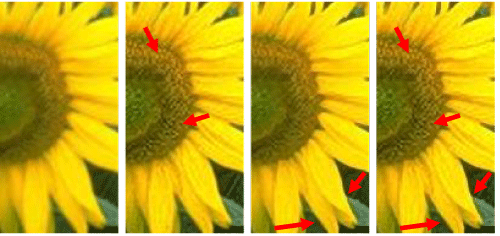Figure 11: The proposed EDUMS method implemented with different gain ratios. (a) Source LR image using 2X bicubic upscaling. (b) EDUMS is weak (Gedums1=1, Gedums2=2) at middle frequencies; the detail stretch (Gdts1=5, Gdts2=3) is strong at high frequencies. The results show fine details and soft edges. (c) EDUMS is strong (Gedums1=3, Gedums2=5) at middle frequencies; the detail stretch (Gdts1=2, Gdts2=1) is weak at high frequencies. The edges become clear, and the details are soft. (d) Both EDUMS (Gedums1=3, Gedums2=5) and the detail stretch (Gdts1=5, Gdts2=3) are strong. The edges and details are vivid.